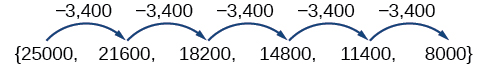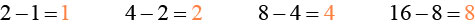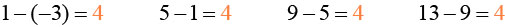13.2 Arithmetic sequences

 Page 1 / 8
In this section, you will:
• Find the common difference for an arithmetic sequence.
• Write terms of an arithmetic sequence.
• Use a recursive formula for an arithmetic sequence.
• Use an explicit formula for an arithmetic sequence.

Companies often make large purchases, such as computers and vehicles, for business use. The book-value of these supplies decreases each year for tax purposes. This decrease in value is called depreciation. One method of calculating depreciation is straight-line depreciation, in which the value of the asset decreases by the same amount each year.

As an example, consider a woman who starts a small contracting business. She purchases a new truck for $25,000. After five years, she estimates that she will be able to sell the truck for$8,000. The loss in value of the truck will therefore be $17,000, which is$3,400 per year for five years. The truck will be worth $21,600 after the first year;$18,200 after two years; $14,800 after three years;$11,400 after four years; and \$8,000 at the end of five years. In this section, we will consider specific kinds of sequences that will allow us to calculate depreciation, such as the truck’s value.

Finding common differences

The values of the truck in the example are said to form an arithmetic sequence because they change by a constant amount each year. Each term increases or decreases by the same constant value called the common difference of the sequence. For this sequence, the common difference is –3,400.The sequence below is another example of an arithmetic sequence. In this case, the constant difference is 3. You can choose any term    of the sequence    , and add 3 to find the subsequent term.Arithmetic sequence

An arithmetic sequence    is a sequence that has the property that the difference between any two consecutive terms is a constant. This constant is called the common difference    . If ${a}_{1}$ is the first term of an arithmetic sequence and $d$ is the common difference, the sequence will be:

$\left\{{a}_{n}\right\}=\left\{{a}_{1},{a}_{1}+d,{a}_{1}+2d,{a}_{1}+3d,...\right\}$

Finding common differences

Is each sequence arithmetic? If so, find the common difference.

1. $\left\{1,2,4,8,16,...\right\}$
2. $\left\{-3,1,5,9,13,...\right\}$

Subtract each term from the subsequent term to determine whether a common difference exists.

1. The sequence is not arithmetic because there is no common difference.2. The sequence is arithmetic because there is a common difference. The common difference is 4.If we are told that a sequence is arithmetic, do we have to subtract every term from the following term to find the common difference?

No. If we know that the sequence is arithmetic, we can choose any one term in the sequence, and subtract it from the subsequent term to find the common difference.

Is the given sequence arithmetic? If so, find the common difference.

The sequence is arithmetic. The common difference is $–2.$

Is the given sequence arithmetic? If so, find the common difference.

The sequence is not arithmetic because $3-1\ne 6-3.$

Writing terms of arithmetic sequences

Now that we can recognize an arithmetic sequence, we will find the terms if we are given the first term and the common difference. The terms can be found by beginning with the first term and adding the common difference repeatedly. In addition, any term can also be found by plugging in the values of $n$ and $d$ into formula below.

A laser rangefinder is locked on a comet approaching Earth. The distance g(x), in kilometers, of the comet after x days, for x in the interval 0 to 30 days, is given by g(x)=250,000csc(π30x). Graph g(x) on the interval [0, 35]. Evaluate g(5)  and interpret the information. What is the minimum distance between the comet and Earth? When does this occur? To which constant in the equation does this correspond? Find and discuss the meaning of any vertical asymptotes.
The sequence is {1,-1,1-1.....} has
how can we solve this problem
Sin(A+B) = sinBcosA+cosBsinA
Prove it
Eseka
Eseka
hi
Joel
June needs 45 gallons of punch. 2 different coolers. Bigger cooler is 5 times as large as smaller cooler. How many gallons in each cooler?
7.5 and 37.5
Nando
find the sum of 28th term of the AP 3+10+17+---------
I think you should say "28 terms" instead of "28th term"
Vedant
the 28th term is 175
Nando
192
Kenneth
if sequence sn is a such that sn>0 for all n and lim sn=0than prove that lim (s1 s2............ sn) ke hole power n =n
write down the polynomial function with root 1/3,2,-3 with solution
if A and B are subspaces of V prove that (A+B)/B=A/(A-B)
write down the value of each of the following in surd form a)cos(-65°) b)sin(-180°)c)tan(225°)d)tan(135°)
Prove that (sinA/1-cosA - 1-cosA/sinA) (cosA/1-sinA - 1-sinA/cosA) = 4
what is the answer to dividing negative index
In a triangle ABC prove that. (b+c)cosA+(c+a)cosB+(a+b)cisC=a+b+c.
give me the waec 2019 questionsByByBy Edward BitonByByBy Rhodes